# 用深度学习识别海洋生物？飞桨轻松完成挑战！

2020/01/06 19:3101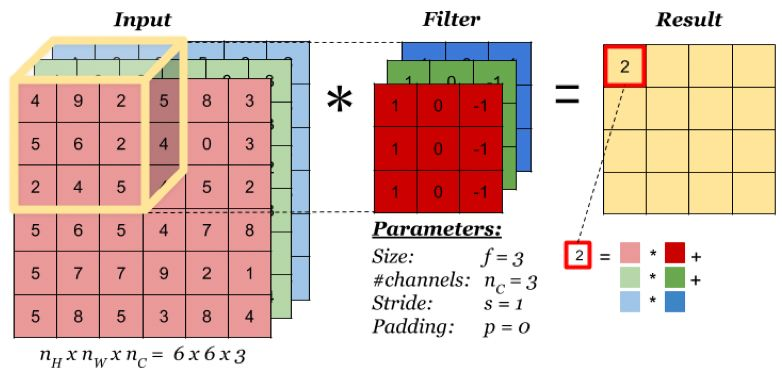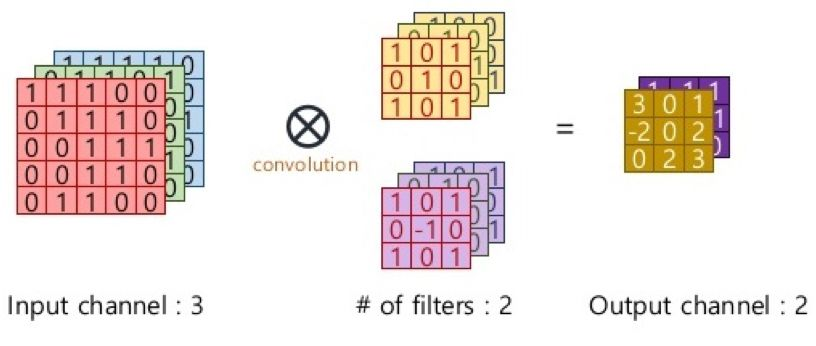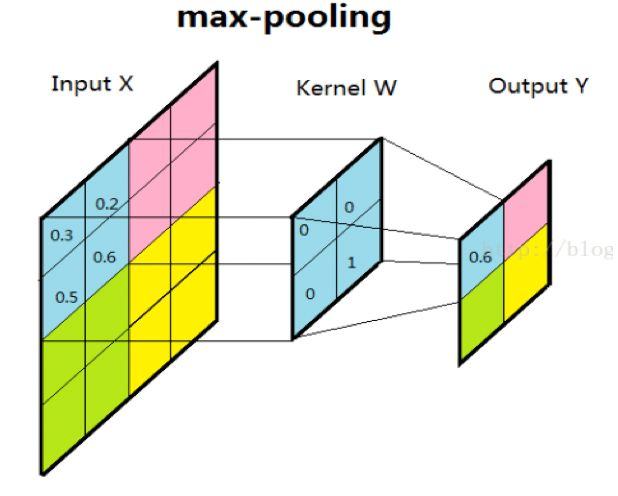02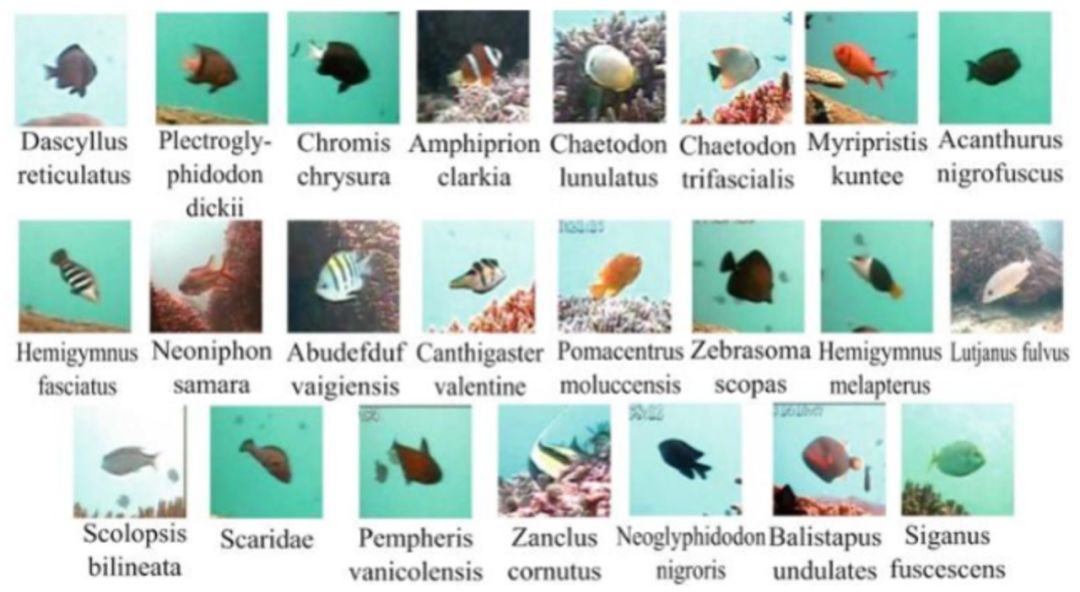#导入必要的包
import os
import numpy as np
from PIL import Image
import paddle.fluid as fluid
from multiprocessing import cpu_count
import matplotlib.pyplot as plt
def data_mapper(sample):
img_path, label = sample
#进行图片的读取，由于数据集的像素维度各不相同，需要进一步处理对图像进行变换
resize_size=47,
crop_size=47,
is_color=True,
is_train=True)
img= img.flatten().astype('float32')/255.0
return img, label

def data_r(file_list,buffered_size=1024):
with open(file_list, 'r') as f:
lines = [line.strip() for line inf]
for line in lines:
img_path, lab =line.strip().split('\t')
yield img_path, int(lab)


BATCH_SIZE = 64
BUF_SIZE=512
#构造训练数据提供器
train_r=data_r(file_list=TRAIN_LIST_PATH)
batch_size=BATCH_SIZE)
#构造测试数据提供器
eval_r = data_r(file_list=EVAL_LIST_PATH)


（1）模型定义

def convolutional_neural_network(img):
# 第一个卷积-池化层
conv1=fluid.layers.conv2d(input=img,       #输入图像
num_filters=20,  #卷积核数量，它与输出的通道相同
filter_size=5,   #卷积核大小
act="relu")      #激活函数
pool1 = fluid.layers.pool2d(
input=conv1,                     #输入
pool_size=2,                     #池化核大小
pool_type='max',                 #池化类型
pool_stride=2)                   #池化步长
conv_pool_1 = fluid.layers.batch_norm(pool1)
# 第二个卷积-池化层
conv2=fluid.layers.conv2d(input=conv_pool_1,
num_filters=50,
filter_size=5,
act="relu")
pool2 = fluid.layers.pool2d(
input=conv2,
pool_size=2,
pool_type='max',
pool_stride=2,
global_pooling=False)
conv_pool_2 = fluid.layers.batch_norm(pool2)
# 第三个卷积-池化层
conv3=fluid.layers.conv2d(input=conv_pool_2,
num_filters=50,
filter_size=5,
act="relu")
pool3 = fluid.layers.pool2d(
input=conv3,
pool_size=2,
pool_type='max',
pool_stride=2,
global_pooling=False)
# 以softmax为激活函数的全连接输出层，23类鱼，所以size=23
prediction = fluid.layers.fc(input=pool3,
size=23,
act='softmax')
return prediction


image = fluid.data(name='image',shape=[None,3, 47, 47], dtype='float32')
label = fluid.data(name='label',shape=[None，1], dtype='int64')


predict=convolutional_neural_network(image)


（2）损失函数

cost =fluid.layers.cross_entropy(input=predict, label=label)
avg_cost = fluid.layers.mean(cost)
accuracy =fluid.layers.accuracy(input=predict, label=label)


test_program=fluid.default_main_program().clone(for_test=True)


（3）优化方法

optimizer = fluid.optimizer.Adam(learning_rate=0.0001)# Adam是一阶基于梯度下降的算法，基于自适应低阶矩估计该函数实现了自适应矩估计优化器
optimizer.minimize(avg_cost)


• fluid.default_startup_program：定义了创建模型参数，输入输出，以及模型中可学习参数的初始化等各种操作，由框架自动生成，使用时无需显示地创建；

• fluid.default_main_program：定义了神经网络模型，前向反向计算，以及优化算法对网络中可学习参数的更新，使用Fluid的核心就是构建起 default_main_program。

use_cuda = True
place = fluid.CUDAPlace(0) if use_cudaelse fluid.CPUPlace()#定义训练设备为CPU或GPU
exe = fluid.Executor(place)
exe.run(fluid.default_startup_program())     #执行参数初始化操作


feeder= fluid.DataFeeder(place=place, feed_list=[image, label])


EPOCH_NUM = 20
for pass_id in range(EPOCH_NUM):
train_cost = 0
for batch_id, data in enumerate(train_reader()):
train_cost, train_acc = exe.run(
program=fluid.default_main_program(),
feed=feeder.feed(data),
fetch_list=[avg_cost,accuracy])
if batch_id % 100 == 0:
print("\nPass %d, Step %d,Cost %f, Acc %f" %
(pass_id, batch_id, train_cost,train_acc))
test_accs = []
test_costs = []
# 每训练一轮 进行一次测试
for batch_id, data in enumerate(eval_reader()):
test_cost, test_acc = exe.run(program=test_program,  、
feed=feeder.feed(data),
fetch_list=[avg_cost, accuracy]
test_accs.append(test_acc)
test_costs.append(test_cost)
test_cost = (sum(test_costs) / len(test_costs))
test_acc = (sum(test_accs) / len(test_accs))           平均准确率
print('Test:%d, Cost:%0.5f, ACC:%0.5f' % (pass_id, test_cost, test_acc))


   model_save_dir ="/home/aistudio/work/model"
if not os.path.exists(model_save_dir):
os.makedirs(model_save_dir)
# 保存训练的模型，executor 把所有相关参数保存到 dirname 中
fluid.io.save_inference_model(model_save_dir,#保存模型的路径
['image'],    #预测需要 feed 的数据
[predict],    #保存预测结果的变量
exe)         #executor 保存预测模型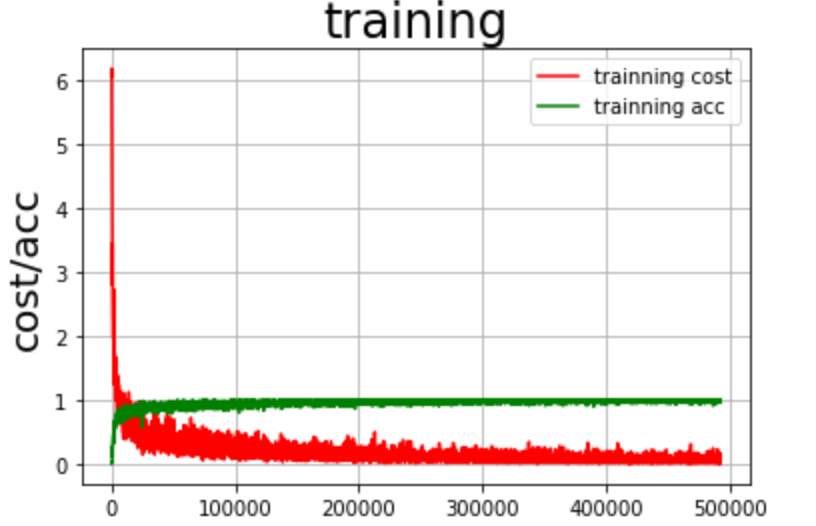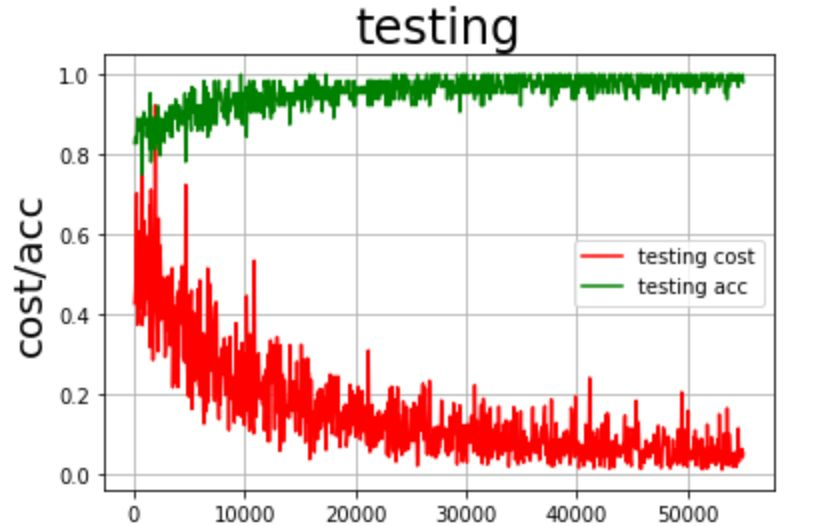# 图片预处理
im = Image.open(file)
im = im.resize((47, 47), Image.ANTIALIAS)
im = np.array(im).reshape(1, 3, 47, 47).astype(np.float32)
im = im / 255.0
return im


#with fluid.scope_guard(inference_scope):
#从指定目录中加载预测模型
[inference_program,# 预测用的program
feed_target_names,# 是一个str列表，它包含需要在推理 Program 中提供数据的变量的名称。
img = Image.open(infer_img)
plt.imshow(img)   #根据数组绘制图像
plt.show()        #显示图像

# 开始预测
results = infer_exe.run(
inference_program,                     #运行预测程序
feed={feed_target_names: image},#喂入要预测的数据
fetch_list=fetch_targets)              #得到推测结果
print('results',results)
label_list = ["fish_21", "fish_19","fish_15", "fish_20","fish_1","fish_5","fish13","fish2","fish4","fish_14","fish_16","fish_6","fish_18","fish_17","fish_22","fish_8","fish_3","fish_23","fish_9","fish_7","fish_12","fish_11","fish_10"]
print("infer results: %s" % label_list[np.argmax(results)])得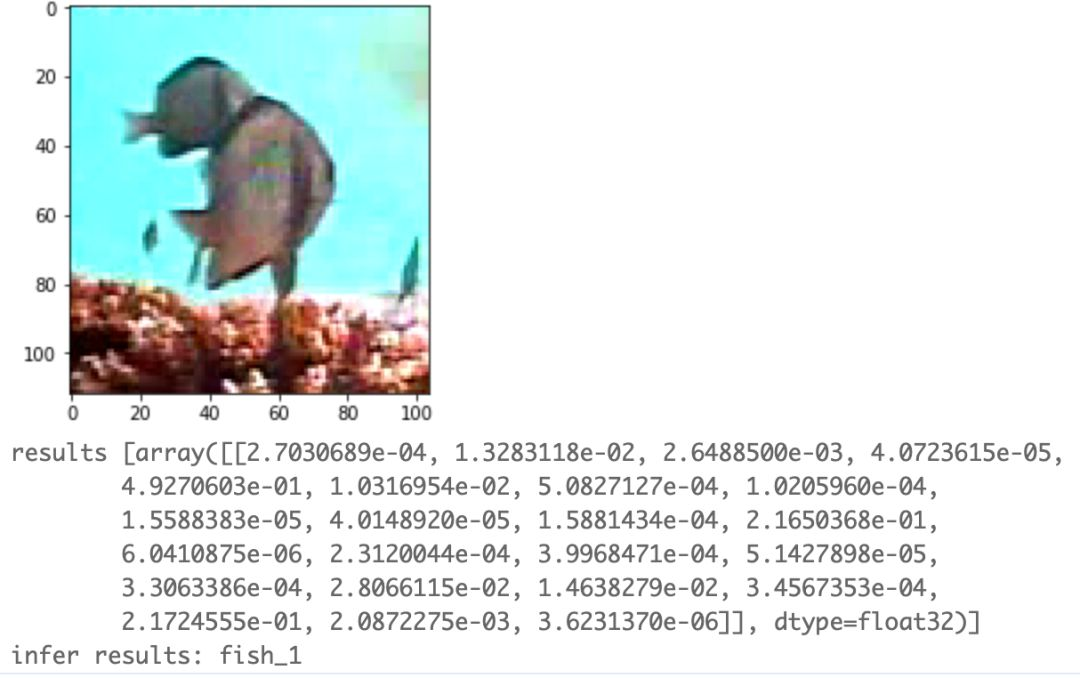https://aistudio.baidu.com/aistudio/projectdetail/155063 可在页面中找到对应实践代码。

https://aistudio.baidu.com/aistudio/course/introduce/996，进行学习

GitHub地址：### 作者的其它热门文章

0
0 收藏

0 评论
0 收藏
0